### MODEL Statement

MODEL model-options ;

The MODEL statement specifies details about the correlation model that you use in the kriging system for prediction. The specified model is used in the kriging system defined by the most previous PREDICT statement. You can specify a semivariogram or covariance model in three ways:

• You specify the required parameters SCALE, RANGE, FORM, and SMOOTH (if you specify the MATERN form), and possibly the optional parameters NUGGET, ANGLE, and RATIO, explicitly in the MODEL statement.

• You specify an MDATA= data set. This data set contains variables that correspond to the required parameters SCALE, RANGE, FORM and SMOOTH (if you specify the MATERN form), and optionally variables for the NUGGET, ANGLE, and RATIO parameters.

• You can specify an input item store in the RESTORE statement. The item store contains one or more correlation models for one or more direction angles. You can specify these models in the STORESELECT option of the MODEL statement to perform a prediction task.

The three methods are mutually exclusive: you specify all parameters explicitly, they are all are read from the MDATA= data set, or you select a model and its parameters from an input item store.

Table 49.3 summarizes the options available in the MODEL statement.

Table 49.3: MODEL Statement Options

Option

Description

Specifies the angle of the major axis

Specifies the functional form (type)

Specifies the input data set containing parameter values

Specifies the nugget effect for the model

Allows values for the power model exponent parameter outside the range of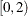Specifies the range parameter

Specifies the ratio of the length of the minor axis

Specifies the scale parameter

Gives the singularity criterion for solving kriging systems

Specifies the smoothness parameter

Uses the information from an input item store

You can use the following model-options with the MODEL statement:

ANGLE=angle
ANGLE=(angle1, …, anglek)

specifies the angle of the major axis for anisotropic models, measured in degrees clockwise from the N-S axis. The default is ANGLE=0.

In the case of a nested semivariogram model with k nestings, you have the following two ways to specify the anisotropy major axis: you can specify only one angle which is then applied to all nested forms, or you can specify one angle for each of the k nestings.

Note: The syntax makes it possible to specify different angles for different forms of the nested model, but this practice is rarely used.

FORM=form
FORM=(form1, …, formk)

specifies the functional form (type) of the semivariogram model. Use the syntax with the single form to specify a non-nested model. Use the syntax with forms formi,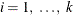, to specify a nested model with k structures. Each of the forms can be any of the following:

CUBIC | EXPONENTIAL | GAUSSIAN | MATERN |
PENTASPHERICAL | POWER | SINEHOLEEFFECT | SPHERICAL
CUB | EXP | GAU | MAT | PEN | POW | SHE | SPH

Usage examples follow.

For example, the syntax

FORM=GAU


specifies a model with a single Gaussian structure. Also, the syntax

FORM=(EXP,SHE,MAT)


specifies a nested model with an exponential, a sine hole effect, and a Matérn structure. Finally

FORM=(EXP,EXP)


specifies a nested model with two structures both of which are exponential.

Note: In the documentation, models are named either by using their full names or by using the first three letters of their structures. Also, the names of different structures in a nested model are separated by a hyphen (-). According to this convention, the previous examples illustrate how to specify a GAU, an EXP-SHE-MAT, and an EXP-EXP model, respectively, with the FORM= option.

All the supported model forms have two parameters specified by the SCALE= and RANGE= options, except for the MATERN model which has a third parameter specified by the SMOOTH= option. A FORM= value is required, unless you specify the MDATA= option or the STORESELECT option.

Computation of the MATERN covariance is numerically demanding. As a result, predictions that use Matérn covariance structures can be time-consuming.

See the section Theoretical Semivariogram Models for details about how the FORM= forms are determined.

MDATA=SAS-data-set

specifies the input data set that contains parameter values for the covariance or semivariogram model. The MDATA= option cannot be combined with any of the FORM= or STORESELECT options.

The MDATA= data set must contain variables named SCALE, RANGE, and FORM, and it can optionally contain variables NUGGET, ANGLE, and RATIO. If you specify the MATERN form, then you must also include a variable named SMOOTH in the MDATA= data set.

The FORM variable must be a character variable, and it can assume only the values allowed in the explicit FORM= syntax described previously. The RANGE, SCALE and SMOOTH variables must be numeric. The optional variables ANGLE, RATIO, and NUGGET must also be numeric if present.

The number of observations present in the MDATA= data set corresponds to the level of nesting of the covariance or semivariogram model. For example, to specify a non-nested model that uses a spherical covariance, an MDATA= data set might be given by the following statement:

data md1;
input scale range form $; datalines; 25 10 SPH run;  The PROC KRIGE2D statement to use the MDATA= specification is of the form shown in the following: proc krige2d data=...; predict var=....; model mdata=md1; run;  This is equivalent to the following explicit specification of the covariance model parameters: proc krige2d data=...; predict var=....; model scale=25 range=10 form=sph; run;  The following MDATA= data set is an example of an anisotropic nested model: data md1; input scale range form$ nugget angle ratio;
datalines;
20 8 SPH  5 35 0.7   .
12 3 MAT  5 0  0.8 2.8
4  1 GAU  5 45 0.5   .
;


This is equivalent to the following explicit specification of the covariance model parameters:

proc krige2d data=...;
predict var=....;
model scale=(20,12,4) range=(8,3,1) form=(SPH,MAT,GAU)
angle=(35,0,45) ratio=(0.7,0.8,0.5) nugget=5 smooth=2.8;
run;


This example is somewhat artificial in that it is usually hard to detect different anisotropy directions and ratios for different nestings by using an empirical semivariogram. Note: The NUGGET variable value is the same for all nestings. This is always the case; the nugget effect is a single additive term for all models. For further details, see the section The Nugget Effect.

The example also shows that if you specify a MATERN form in the nested model, then the SMOOTH variable must be specified for all nestings in the MDATA= data set. You simply specify the SMOOTH value as missing for nestings other than MATERN.

NUGGET=number

specifies the nugget effect for the model. The nugget effect is due to a discontinuity in the semivariogram as determined by plotting the sample semivariogram. For details, see the section The Nugget Effect and Chapter 102: The VARIOGRAM Procedure. For models without any nugget effect, this option is left out; the default is NUGGET=0.

POWNOBOUND

specifies that values for the power model exponent parameter outside the range ofbe allowed. The POWNOBOUND option applies only when you specify a power form in the MODEL statement.

Power models yield permissible covariance models only when the exponent parameter is nonnegative and less than 2. By default, PROC KRIGE2D produces an error if you specify a negative power exponent or one that is equal to or larger than 2 in the RANGE= option of the MODEL statement.

See the section The Power Semivariogram Model for more details about the power model form and its exponent parameter.

RANGE=range
RANGE=(range1, …, rangek)

specifies the range parameter in semivariogram models. If you have anisotropy, you must specify the range of the major anisotropy axis, or the range of the minor anisotropy axis for any zonal components. In the case of a nested semivariogram model with k nestings, you must specify a range for each nested structure.

The range parameter has units of distance, and it is related to the correlation scale for the underlying spatial process.

Note: If you specify this parameter for a power model, then it does not correspond to a range. For power models, the parameter you specify in the RANGE option is a dimensionless power exponent whose value must range within [0,2) so that the power model is a valid semivariance function. See also the POWNOBOUND option of the MODEL statement.

See the section Theoretical Semivariogram Models for details about how the RANGE= values are determined.

RATIO=ratio
RATIO=(ratio1, …, ratiok)

specifies the ratio of the length of the minor axis to the length of the major axis for anisotropic models. The value of the RATIO= option must be between 0 and 1. An exception is the case of zonal anisotropy, where the ratio of zonal components must be designated by a very large number for the RATIO= option. For further details, see the section Zonal Anisotropy.

In the case of a nested semivariogram model with k nestings, you can specify a ratio for each nesting. The default is RATIO=1.

SCALE=scale
SCALE=(scale1, …, scalek)

specifies the scale parameter in semivariogram models. In the case of a nested semivariogram model with k nestings, you must specify a scale for each nesting.

The scale parameter is the multiplicative factor in all supported models; it has the same units as the variance of the VAR= variable in the preceding PREDICT statement.

In power models the SCALE= parameter does not correspond to a sill because the power model has no sill. Instead, PROC KRIGE2D uses the SCALE= option to designate the slope (or scaling factor) in power model forms. The power model slope has the same variance units as the VAR= variable.

See the section Theoretical Semivariogram Models for details about how the SCALE= values are determined.

SINGULAR=number

gives the singularity criterion for solving kriging systems. The larger the value of the SINGULAR= option, the easier it is for a kriging system to be declared singular. The default is SINGULAR=1E–7. See the section Ordinary Kriging for more detailed information.

SMOOTH=smooth
SMOOTH=(smooth1, …, smoothm)

specifies the smoothness parameterin the Matérn type of semivariance structures. The special caseis equivalent to the exponential model, whereasgives the Gaussian model.

When you specify m different MATERN forms in the FORM= option, you must also provide m smoothness values in the SMOOTH option. If you must specify more than one smoothness value, the values are assigned sequentially to the MATERN nestings in the order the nestings are specified. If you specify more smoothness values than necessary, then values in excess are ignored.

STORESELECT(ssel-options)
SSEL(ssel-options)

specifies that information from an input item store be used for the prediction. You cannot combine the STORESELECT option with any of the FORM= or MDATA= options. The STORESELECT option has the following ssel-options:

TYPE=field-type

specifies whether to perform isotropic or anisotropic prediction. You can choose the field-type from one of the following:

ISO

specifies an isotropic field for the prediction.

ANIGEO | GEO

specifies a field with geometric anisotropy for the prediction.

ANIZON(zonal-form1, …, zonal-formn)
ZON(zonal-form1, …, zonal-formn)

specifies a field with zonal anisotropy for the prediction. Each zonal-formi,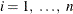, can be any of the following:

CUB | EXP | GAU | MAT | PEN | POW | SHE | SPH

Each zonal-formi,, is a structure in the purely zonal component of the correlation model in the direction angle of the minor anisotropy axis. For this reason, when you specify the TYPE=ANIZON suboption you must also specify the nonzonal component of the correlation model in the MODEL= suboption of the STORESELECT option.

Assume the nonzonal component has k structures; these are common across all directions and each one has the same scale in all directions. In that sense, you use the TYPE=ANIZON suboption to specify only the n zonal anisotropy structures of an input store ()-structure nested model in the direction angle of the minor anisotropy axis.

Given this specification,must be up to the maximum number of nested model structures that is supported by the item store. See also the MODEL= suboption of the STORESELECT option.

In conclusion, you can use an input item store for prediction with zonal anisotropy if you know that every structure in the nonzonal model component has the same scale across all directions. When this condition does not apply for the item store models, specify the model parameters explicitly in the MODEL statement. For more details, see the examples in the section Zonal Anisotropy.

Computation of the MATERN covariance is numerically demanding. As a result, predictions that use Matérn covariance structures can be time-consuming.

If you omit the TYPE= option, the default behavior is TYPE=ISO when the input item store contains information for only one angle or for the omnidirectional case. If you specify an item store with information for more than one direction, then the default behavior is TYPE=ANIGEO.

When you specify TYPE=ISO to request isotropic analysis in the presence of an item store with information for multiple directions, you must specify the ANGLEID= suboption of the STORESELECT option with one argument. This argument specifies which of the direction angles information to use for the isotropic analysis.

When you indicate the presence of anisotropy with the TYPE=ANIGEO or TYPE=ANIZON suboptions of the STORESELECT option, the following conditions apply:

• You must specify the ANGLEID= suboption of the STORESELECT option to designate the major and minor anisotropy axes. See the ANGLEID= suboption of the STORESELECT option for details.

• For TYPE=ANIGEO, ensure that you have the same scale in all anisotropy directions.

• For TYPE=ANIZON, ensure that the nonzonal component scale is the same in all anisotropy directions.

If you import a nested model, these rules also apply to each one of the nested structures.

• Model ranges in the major anisotropy axis must be longer than ranges in the minor anisotropy axis.

• Any Matérn covariance structure must maintain its smoothness parameter value in all anisotropy directions.

ANGLEID=angleid1
ANGLEID=(angleid1, angleid2)

specifies which direction angles in the input item store be used for prediction. The angles are identified by the corresponding number in the AngleID column of the Store Models Information table, or by the AngleID parameter in the table title when you specify the INFO(DETAILS) option in the RESTORE statement.

If you request isotropic prediction in the TYPE= suboption of the STORESELECT option and the item store has omnidirectional contents or information about only one angle, then the ANGLEID= option is ignored. The prediction input comes from the omnidirectional information. In the case of a single angle, you still perform isotropic prediction and the model parameters are provided by the model in the single direction angle in the item store. However, if the item store contains information for more than one angle, then you must specify one angle ID in angleid1. The model information from the corresponding angle is then used in your isotropic prediction.

When you specify an anisotropic prediction in the TYPE= option of the STORESELECT option, you need to have information about two perpendicular direction angles. One of them is the major and the other is the minor anisotropy axis. You must always specify the major anisotropy axis angle ID in angleid1 and the minor anisotropy axis angle ID in angleid2. This means that the range parameters of the model forms in the angle designated by the angleid1 need to be larger than the corresponding ranges of the forms in the angle designated by the angleid2. Conveniently, if the item store has only two angles, then you only need to specify the ID angleid1 of the major anisotropy axis angle. If the item store has only one angle, then you cannot perform anisotropic prediction with input from the item store.

Note: You can perform geometric anisotropic analysis even if the item store does not contain information about a direction that is perpendicular to the one specified by angleid1. This is possible due to the geometry of the ellipse. In particular, when you specify the major axis with angleid1 and an angle ID for a second direction with a corresponding smaller range, then PROC KRIGE2D automatically computes the minor anisotropy axis range and the necessary range ratio parameter.

Anisotropic analysis is not possible when you specify instances of the same angle in the input item store. It is possible that PROC VARIOGRAM produces an item store in which two or more directions can be the same if their corresponding correlation models were obtained for different angle tolerances or bandwidths in the VARIOGRAM procedure. Consequently, you cannot specify anisotropic prediction if the input store contains only two angles that are the same or if you specify angleid1 and angleid2 that correspond to equal angles.

MODEL=form
MODEL=(form1, …, formk)

specifies the theoretical semivariogram model selection to use for the prediction. Use any combination of one, two, or three forms to describe a model in the input item store because up to three nested structures are supported. Each formi,, can be any of the following:

CUB | EXP | GAU | MAT | PEN | POW | SHE | SPH

Computation of the MATERN covariance is numerically demanding. As a result, predictions that use Matérn covariance structures can be time-consuming.

All fitted models that are stored in the input item store contain information about their component parameters and also about the nugget effect if any. The KRIGE2D procedure retrieves this information when you make a model selection in the MODEL= option, and you do not need to individually specify a nugget effect or any other parameter of the model.

By default, the model that is ranked first among the models for a given angle in the item store is used for the prediction task. If more than one model is available in the item store, then you can specify the MODEL= option to use a different model for the prediction.

In an anisotropic prediction, the default selection is the model that is ranked first in the direction angle of the major anisotropy axis. If you specify the TYPE=ANIGEO option, then a model that consists of identical structures needs to be present in the selected minor anisotropy axis angle in the item store. If you specify the TYPE=ANIZON option, then a model with the exact same first k structures must be present in the selected minor anisotropy axis angle, and it must feature at least one more structure as a zonal component. The zonal component is specified separately in the TYPE=ANIZON suboption of the STORESELECT option. Consequently, remember that in zonal anisotropy the MODEL= suboption designates only the nonzonal component of the correlation model in the minor anisotropy axis direction. In all, if there are k common structures and n structures in the purely zonal component, thenmust be up to the maximum number of nested model structures that is supported by the item store.

In comparison to the other two ways of specifying a correlation model in PROC KRIGE2D, the STORESELECT option is quite different because you can avoid explicit specification of all parameter values of a model. When you specify the STORESELECT option, then the corresponding scale, range, nugget effect, and smoothness (if appropriate) parameter values are invoked as saved attributes of the model that you select from the item store.

In the case of anisotropy, you specify the angles indirectly with the ANGLEID= option of the STORESELECT option, and the ratios are computed implicitly by using the selected model ranges. Explore how to specify valid anisotropical models imported from an input item store with the two examples that follow.

In the first example, assume the input item store InStoreGeo contains exponential models in the angles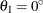,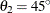, and. You know in advance that all models have the same scale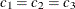across these directions and that the respective ranges are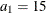,, and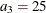in distance units. Hence, you have a case of geometric anisotropy where the major anisotropy axis is in the direction of angleand the minor anisotropy axis is in the direction of angle. The following statements in PROC KRIGE2D use the information in the item store InStoreGeo to perform simulation under the assumption of geometric anisotropy:

proc krige2d data=...;
restore in=InStoreGeo;
predict var=....;
model storeselect(model=exp type=anigeo angleid=(3,1));
run;


For the second example, assume a case of zonal anisotropy. Consider the input item store InStoreZon, which contains models in the two angles,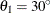and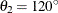. Specifically, inyou have an exponential-spherical model: the exponential structure has scaleand range; the spherical structure has scale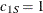and range. In direction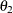you have an exponential model with scaleand range. Hence, the zonal anisotropy major axis is in the direction of the lowest total variance, which is in angle; then, the minor axis is in the direction of angle. The following statements in PROC KRIGE2D use the information in the store InStoreZon to perform prediction under the assumption of zonal anisotropy:

proc krige2d data=...;
restore in=InStoreZon;
predict var=....;
model storeselect(model=exp type=anizon(sph) angleid=(2,1));
run;